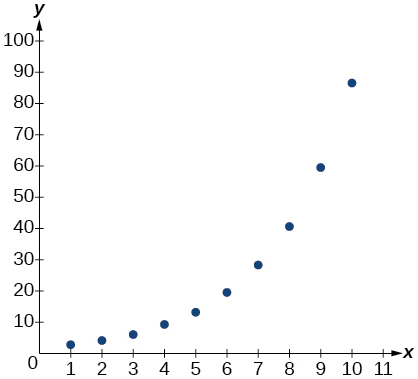# 6.8 Fitting exponential models to data  (Page 11/12)

 Page 11 / 12

The population of a city is modeled by the equation $\text{\hspace{0.17em}}P\left(t\right)=256,114{e}^{0.25t}\text{\hspace{0.17em}}$ where $\text{\hspace{0.17em}}t\text{\hspace{0.17em}}$ is measured in years. If the city continues to grow at this rate, how many years will it take for the population to reach one million?

about $\text{\hspace{0.17em}}5.45\text{\hspace{0.17em}}$ years

Find the inverse function $\text{\hspace{0.17em}}{f}^{-1}\text{\hspace{0.17em}}$ for the exponential function $\text{\hspace{0.17em}}f\left(x\right)=2\cdot {e}^{x+1}-5.$

Find the inverse function $\text{\hspace{0.17em}}{f}^{-1}\text{\hspace{0.17em}}$ for the logarithmic function $\text{\hspace{0.17em}}f\left(x\right)=0.25\cdot {\mathrm{log}}_{2}\left({x}^{3}+1\right).$

${f}^{-1}\left(x\right)=\sqrt{{2}^{4x}-1}$

## Exponential and Logarithmic Models

For the following exercises, use this scenario: A doctor prescribes $\text{\hspace{0.17em}}300\text{\hspace{0.17em}}$ milligrams of a therapeutic drug that decays by about $\text{\hspace{0.17em}}17%\text{\hspace{0.17em}}$ each hour.

To the nearest minute, what is the half-life of the drug?

Write an exponential model representing the amount of the drug remaining in the patient’s system after $\text{\hspace{0.17em}}t\text{\hspace{0.17em}}$ hours. Then use the formula to find the amount of the drug that would remain in the patient’s system after $\text{\hspace{0.17em}}24\text{\hspace{0.17em}}$ hours. Round to the nearest hundredth of a gram.

$f\left(t\right)=300{\left(0.83\right)}^{t};f\left(24\right)\approx 3.43\text{ }\text{ }g$

For the following exercises, use this scenario: A soup with an internal temperature of $\text{\hspace{0.17em}}\text{350°}\text{\hspace{0.17em}}$ Fahrenheit was taken off the stove to cool in a $\text{\hspace{0.17em}}\text{71°F}\text{\hspace{0.17em}}$ room. After fifteen minutes, the internal temperature of the soup was $\text{\hspace{0.17em}}\text{175°F}\text{.}$

Use Newton’s Law of Cooling to write a formula that models this situation.

How many minutes will it take the soup to cool to $\text{\hspace{0.17em}}\text{85°F?}$

about $\text{\hspace{0.17em}}45\text{\hspace{0.17em}}$ minutes

For the following exercises, use this scenario: The equation $\text{\hspace{0.17em}}N\left(t\right)=\frac{1200}{1+199{e}^{-0.625t}}\text{\hspace{0.17em}}$ models the number of people in a school who have heard a rumor after $\text{\hspace{0.17em}}t\text{\hspace{0.17em}}$ days.

How many people started the rumor?

To the nearest tenth, how many days will it be before the rumor spreads to half the carrying capacity?

about $\text{\hspace{0.17em}}8.5\text{\hspace{0.17em}}$ days

What is the carrying capacity?

For the following exercises, enter the data from each table into a graphing calculator and graph the resulting scatter plots. Determine whether the data from the table would likely represent a function that is linear, exponential, or logarithmic.

 x f(x) 1 3.05 2 4.42 3 6.4 4 9.28 5 13.46 6 19.52 7 28.3 8 41.04 9 59.5 10 86.28

exponentialx f(x) 0.5 18.05 1 17 3 15.33 5 14.55 7 14.04 10 13.5 12 13.22 13 13.1 15 12.88 17 12.69 20 12.45

Find a formula for an exponential equation that goes through the points $\text{\hspace{0.17em}}\left(-2,100\right)\text{\hspace{0.17em}}$ and $\text{\hspace{0.17em}}\left(0,4\right).\text{\hspace{0.17em}}$ Then express the formula as an equivalent equation with base e.

$y=4{\left(0.2\right)}^{x};\text{\hspace{0.17em}}$ $y=4{e}^{\text{-1}\text{.609438}x}$

## Fitting Exponential Models to Data

What is the carrying capacity for a population modeled by the logistic equation $\text{\hspace{0.17em}}P\left(t\right)=\frac{250,000}{1\text{\hspace{0.17em}}\text{\hspace{0.17em}}+\text{\hspace{0.17em}}\text{\hspace{0.17em}}499{e}^{-0.45t}}?\text{\hspace{0.17em}}$ What is the initial population for the model?

The population of a culture of bacteria is modeled by the logistic equation $\text{\hspace{0.17em}}P\left(t\right)=\frac{14,250}{1\text{\hspace{0.17em}}\text{\hspace{0.17em}}+\text{\hspace{0.17em}}\text{\hspace{0.17em}}29{e}^{-0.62t}},$ where $\text{\hspace{0.17em}}t\text{\hspace{0.17em}}$ is in days. To the nearest tenth, how many days will it take the culture to reach $\text{\hspace{0.17em}}75%\text{\hspace{0.17em}}$ of its carrying capacity?

about $\text{\hspace{0.17em}}7.2\text{\hspace{0.17em}}$ days

For the following exercises, use a graphing utility to create a scatter diagram of the data given in the table. Observe the shape of the scatter diagram to determine whether the data is best described by an exponential, logarithmic, or logistic model. Then use the appropriate regression feature to find an equation that models the data. When necessary, round values to five decimal places.

#### Questions & Answers

A laser rangefinder is locked on a comet approaching Earth. The distance g(x), in kilometers, of the comet after x days, for x in the interval 0 to 30 days, is given by g(x)=250,000csc(π30x). Graph g(x) on the interval [0, 35]. Evaluate g(5)  and interpret the information. What is the minimum distance between the comet and Earth? When does this occur? To which constant in the equation does this correspond? Find and discuss the meaning of any vertical asymptotes.
Kaitlyn Reply
The sequence is {1,-1,1-1.....} has
amit Reply
circular region of radious
Kainat Reply
how can we solve this problem
Joel Reply
Sin(A+B) = sinBcosA+cosBsinA
Eseka Reply
Prove it
Eseka
Please prove it
Eseka
hi
Joel
June needs 45 gallons of punch. 2 different coolers. Bigger cooler is 5 times as large as smaller cooler. How many gallons in each cooler?
Arleathia Reply
7.5 and 37.5
Nando
find the sum of 28th term of the AP 3+10+17+---------
Prince Reply
I think you should say "28 terms" instead of "28th term"
Vedant
the 28th term is 175
Nando
192
Kenneth
if sequence sn is a such that sn>0 for all n and lim sn=0than prove that lim (s1 s2............ sn) ke hole power n =n
SANDESH Reply
write down the polynomial function with root 1/3,2,-3 with solution
Gift Reply
if A and B are subspaces of V prove that (A+B)/B=A/(A-B)
Pream Reply
write down the value of each of the following in surd form a)cos(-65°) b)sin(-180°)c)tan(225°)d)tan(135°)
Oroke Reply
Prove that (sinA/1-cosA - 1-cosA/sinA) (cosA/1-sinA - 1-sinA/cosA) = 4
kiruba Reply
what is the answer to dividing negative index
Morosi Reply
In a triangle ABC prove that. (b+c)cosA+(c+a)cosB+(a+b)cisC=a+b+c.
Shivam Reply
give me the waec 2019 questions
Aaron Reply

### Read also:

#### Get the best Algebra and trigonometry course in your pocket!

Source:  OpenStax, Algebra and trigonometry. OpenStax CNX. Nov 14, 2016 Download for free at https://legacy.cnx.org/content/col11758/1.6
Google Play and the Google Play logo are trademarks of Google Inc.

Notification Switch

Would you like to follow the 'Algebra and trigonometry' conversation and receive update notifications?ByByBy Lakeima RobertsBy Anonymous UserBy RhodesBy Abishek DevarajBy Edward Biton